Directions for questions 1 to 5: Answer the questions on the basis of the information given below :
Two traders, Rahul and Virat took buying and selling decisions at the end of each trading day. Rahul and Virat, were involved in the buying and selling of RATNA shares over five trading days. At the end of each day, the RATNA share price either went up by Rs 10, or else, it came down by Rs 10. At the beginning of the first day, the RATNA share was priced at Rs 100, while at the end of the fifth day it was priced at Rs 110. The beginning price of RATNA share on a given day was the same as the ending price of the previous day. Rahul and Virat started with the same number of shares and amount of cash, and had enough of both. Below are some additional facts about how Rahul and Virat traded over the five trading days.

Each day, if it was less than or equal to Rs 90, Virat bought 10 shares, all at the closing price.If on any day, the closing price was above Rs. 110, then he sold 10 shares of RATNA.
On the other hand, each day if the price went down, Rahul bought 10 shares at the closing price.if the price went up, he sold 10 shares of RATNA at the closing price.

Question 1 : What would be the maximum possible increase in combined cash balance of Rahul and Virat at the end of the fifth day?
(a) Rs. 4000
(b) Rs. 3700
(c) Rs. 6000
(d) Rs. 5000
(e) Rs. 4700

Question 2 : If Virat sold 10 shares only once during the five days while Rahul sold 10 shares of RATNA on three consecutive days, , what was the price of RATNA at the end of day 3 ?
(a) Rs. 90
(b) Rs. 100
(c) Rs. 110
(d) Rs. 120
(e) Rs. 130

Question 3 : If Rahul ended up with Rs. 100 more cash than Virat at the end of day 5, the difference in the number of shares possessed by Virat and Rahul (at the end of day 5) is
(a)   Rahul had 20 more shares than Virat
(b)   Virat had 10 less shares han Rahul
(c)   Virat had 10 more shares than Rahul
(d)   Rahul had 10 more shares than Virat
(e)   Both had the same number of shares

Question 4 : If Virat ended up with 20 more shares than Rahul at the end of day 5, the price of the share at the end of day 3 is
(a) Rs. 90
(b) Rs. 130
(c)  Rs. 120
(d) Rs. 110
(e) Rs. 100

Question 5 : If Virat ended up with Rs 1300 less cash than Rahul at the end of day 5, the price of RATNA share at the end of day 4 is
(a) Rs. 90
(b) Rs. 100
(c) Rs. 120
(d) Rs. 110
(e) Not uniquely determinable

The order of share prices when both of them could have maximum possible increase in combined cash balance is as follows: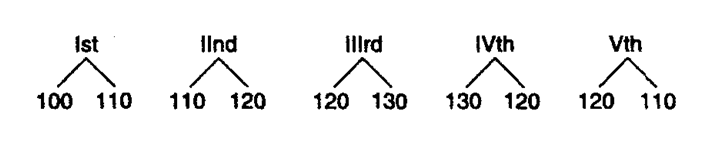Therefore, cash with Rahul at the end of 5th day

= 10 × 110 +10 × 120 +10 × 130 –10 × 120 – 10 × 110 = Rs 1300 and with that of Virat

= 10 × 120 +10 × 130 +10 × 120 = 1200 +1200 +1300 = Rs 3700

Hence, combined increase in cash = 1300 + 3700 = Rs 5000

Rahul sold 10 shares when price of share went up by Rs 10 and Virat sold 10 shares when closing price was above Rs 110. Then, according to the given conditions, the arrangement of opening and closing prices of RATNA shares are as below.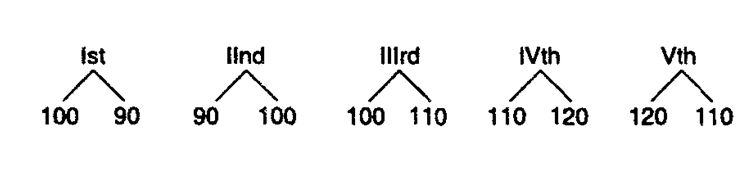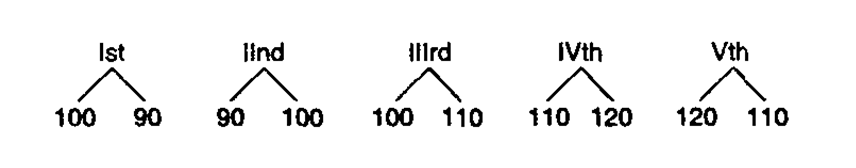Let initial amount with Rahul and Virat is Rs y.

Then, total money with Rahul

= y – 900 + 1000 + 1100 + 1200 – 1100 = y + 1300

Total money with Virat = y + 1200

Therefore, difference between Rahul and Virat is Rs 100 and number of shares with Virat and Rahul is the same.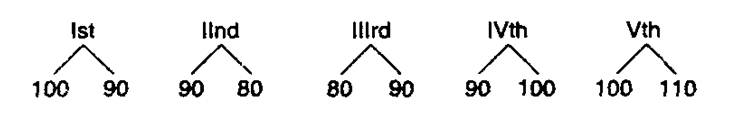Assume that initial number of shares with Rahul and Virat is x.

According the above schedule Rahul buys 10 shares on Ist and IInd days and sells shares of IIIrd, IVth and Vth days.

∴     Total shares with the Rahul = (x – 10)

Virat buys shares only on second day.

∴      Total shares with Virat is (x +10)

∴       Virat had 20 shares more than Rahul.

Hence, price at the end of third day is Rs 90.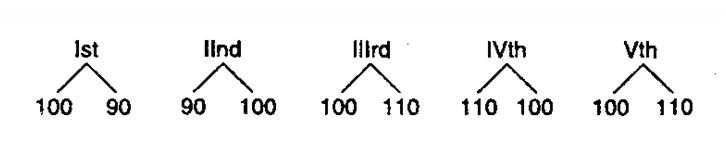Let original money with Rahul and Virat be Rs x

Then, money with Rahul at the end of 5th day

= x – 900 + 1000 + 1100 – 1000 + 1100 = x + 1300

Money with Virat = x

Therefore, Rahul ended up with Rs 1300 more cash than Virat.

Hence, at the end of the 4th day the price of share is Rs 100.